# 2.13 RANDOM INCIDENCE

The Poisson process is one of many stochastic processes that one encounters in urban service systems. The Poisson process is one example of a "point process" in which discrete events (arrivals) occur at particular points in time. For a general point process having its zeroth arrival at time T0 and the remaining arrivals at times T1, T2, T3, . . ., the interarrival times areSuch a stochastic process is fully characterized by the family of joint pdf'sfor all integer values of p and all possible combinations of different n1, n2, . . ., where each ni is a positive integer denoting a particular interarrival time. Maintaining the depiction of a stochastic process at such a general level, although fine in theory, yields an intractable model and one for which the data (to estimate all the joint pdf 's) are virtually impossible to obtain. So, in the study of stochastic processes, one is motivated to make assumptions about this family of pdf's that (1) are realistic for an important class of problems and (2) yield a tractable model.

We wish to consider here the class of point stochastic processes for which the marginal pdf's for all of the interarrival times (Yk) are identical. That is, we assume that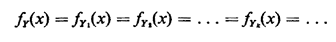Thus, for Yk, if we selected any one of the family of joint pdf's fYn1,Yn2, . . ., Ynp (yn1, yn2, . . . , yk, . . ., ynP) and "integrated out" all variables except yk, we would obtain fY(.). Note that we have said nothing about independence of the Yk's

They need not be mutually independent, pairwise independent, or conditionally independent in any way. For the special case in which the Yk's are mutually independent, the point process is called a renewal process. The Poisson process is a special case of a renewal process, being the only continuous-time renewal process having "no memory." However, the kind of process we are considering can exhibit both memory and dependence among the inter-event times. In fact, the dependence could be so strong that once we know the value of one of the Yk's we might know a great deal (perhaps even the exact values) of any number of the remaining Yk's.

Example 5: Bus Stop

Consider a potential bus passenger arriving at a bus stop. The kth bus arrives Yk time units after the (k - 1)st bus. Here the Yk's are called bus headways. The probabilistic behavior of the Yk's will determine the probability law for the waiting time of the potential passenger (until the next bus arrives). Here it is reasonable to assume that the Yk's are identically distributed but not independent (due to interactions between successive buses). One could estimate the pdf fY(.) simply by gathering data describing bus interarrival times and displaying the data in the form of a histogram. (This same model applies to subways and even elevators in a multielevator building.)

Example 6: Police Patrol

Consider the process of passings of a police patrol car by a residence or business. Successive passings may be identically distributed but not independent. For instance, the patrolling officer may think to himself or herself: "Well, I've passed that address three times during the last two hours, so I won't go by there again until tomorrow."

In situations such as these, for which we know fY(.) or at least the mean and variance of Y, we are often interested in the following problem. An individual, say a potential bus passenger or a homeowner looking for a police patrol car, starts observing the process at a random time, and he or she wishes to obtain the probability law (or at least the mean) of the time he or she must wait until the next arrival occurs. In various applications this time could be the waiting time for a bus, subway, or elevator or the time until arrival of a patrol car. This is said to be a problem of random incidence, since the individual observer is incident to the process at a random time. The random time assumption is important: the time of random incidence of the observer can in no way depend on the past history of actual arrival times in the process.

We now derive the probability law for V, the time from the moment of random incidence until the next arrival occurs. We do this for continuous random variables since the same reasoning applies in the discrete case. The derivation proceeds in stages, first conditioning on W, the length of the interarrival gap entered by random incidence. For instance, the gap in which a potential bus passenger arrives has length equal to the sum of two time intervals: (1) the time between the arrival of the most recent bus and the arrival of the potential passenger, and (2) the time between the passenger's arrival and the arrival of the next bus. We now argue that the probability that the gap entered by random incidence assumes a value between w and w + dw is proportional to both the relative frequency of occurrence of such gaps fY(w)dw and the duration of the gap w. That is,This result says that random incidence favors gaps of longer duration in direct proportion to their duration. The argument for this result is most simply given by example. Given two gap lengths w1 and w2 = 2w1 for which the relative frequencies are identical [fY(w1)dw = fY(w2)dw], then one is twice as likely to enter the gap of length 2w11 compared to the gap of length w1. Or, given the same two gap lengths, w1 and w2 = 2w1, for which the relative frequency of the large gap length is only half that of the smaller [fY(w2)dw = (1/2)fY(w1)dw], we are equally likely to enter either of the two types of gaps; here the doubling of relative frequency for w, "makes up for" the doubling of duration of w2.

Now, given that we have entered a gap of length w by random incidence, we are equally likely to be anywhere within the gap. More precisely, there is a constant probability of being in any interval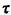to+ h for any fixed h > 0, assuming that [,+ h] is fully contained within the gap. Thus, given w, the time until gap completion (i.e., the time until the next event) has a uniform pdf:The marginal for V, which is what we want, is formed simply by "integrating out" W,which can be expressed in terms of the cdf for Y,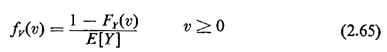Question: Assuming that FY(O) = 0, does this result make intuitive sense for values of v near zero?

Example 5: (continued)

Suppose that buses maintain perfect headway; that is, they are always T0 minutes apart. Then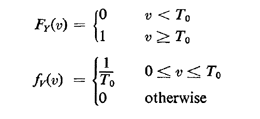That is, the time until the next bus arrives, given random incidence, is uniformly distributed between 0 and T0, with a mean E[V] = T0/2, as we might expect intuitively.

Example 6: (continued)

Suppose that police cars patrol in a completely random manner, with car passings occurring according to a Poisson process with mean rate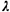passings per day. Then interpassing times are distributed as negative exponential random variables with mean 1/. Hence,as we expect from the no-memory property of Poisson processes.

Example 7. Clumped Buses

Suppose that buses along a particular route are on schedule "half the time" and "clumped" together in pairs the other half of the time. That is, for 50 percent of the day (which 50 percent is unpredictable), the bus headways are exactly T0. For the remaining 50 percent of the day, (crowded) buses arrive in pairs, the time between each pair being 2T0. Note that in this case, given paired buses, half of the bus interarrival times are 2T0 and half are 0 (!), because of zero elapsed time between two paired buses. Thus,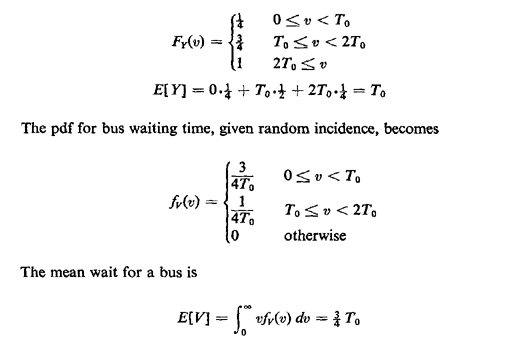which represents a 50 percent increase over the case of perfect headways. (Note that successive bus interarrival times are not independent in this case.)

Sometimes we may only be interested in E[V], the mean time from the moment of random incidence until the next arrival occurs. Or, we may have only partial statistics about Y, perhaps the first few moments, and we desire to obtain at least E[V]. We may compute E[V] simply by conditioning on the length of the gap entered and integrating over all possible gap lengths: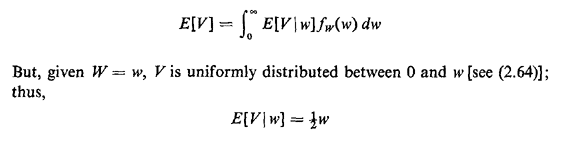Using (2.63), we can now writeThus, the mean time from random incidence until the next event depends only on the mean and variance of the inter-event time Y.It is interesting to examine (2.66) to acquire an understanding of the range of plausible values of E[V]. The minimum possible mean waiting time until the next arrival is (1/2)E[Y], which occurs for a "perfectly scheduled" system (i.e.,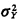= 0). For systems with temporal irregularity comparable to that of the Poisson process, namely those for which the standard deviation of interarrival timesequals the mean E[Y], the waiting time E[V] is equal to the mean E[Y]. Thus, for such systems one incurs the same average wait arriving at random as an observer arriving immediately after the most recent event (e.g., bus). Intuitively, in this case one half the mean wait is due to the average spacing between successive buses and the other half is due to uncertainties (i.e., randomness) in the arrival process. For systems with irregularity greater than the Poisson process, namely those for which> E[Y], the mean wait of the observer can assume any value greater than E[Y]; here, somewhat surprisingly, one waits longer (on the average) arriving at a random time compared to arriving just after the most recent event. An example of such a situation would be arriving at the turnstiles of a sports stadium, say Yankee Stadium in New York, and waiting for the next sports fan to pass through the turnstile. The wait is likely to be very small (say in the order of seconds) if one arrives just after the arrival of a random fan (thereby guaranteeing that a baseball game is about to be played) compared to arriving at some random time during the year (in which case the wait is likely to be quite long if one arrives, say, in December).